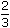# Aptitude - Percentage

### Exercise :: Percentage - General Questions

6.

If 20% of a = b, then b% of 20 is the same as:

 A. 4% of a B. 5% of a C. 20% of a D. None of these

Explanation:

 20% of a = b20 a = b. 100b% of 20 =b x 20=20 a x 1 x 20= 4 a = 4% of a. 100 100 100 100

7.

In a certain school, 20% of students are below 8 years of age. The number of students above 8 years of age isof the number of students of 8 years of age which is 48. What is the total number of students in the school?

 A. 72 B. 80 C. 120 D. 150 E. 100

Explanation:

Let the number of students be x. Then,

Number of students above 8 years of age = (100 - 20)% of x = 80% of x.80% of x = 48 + 2 of 48 380 x = 80 100x = 100.

Video Explanation: https://youtu.be/yPfocU6DA2M

8.

Two numbers A and B are such that the sum of 5% of A and 4% of B is two-third of the sum of 6% of A and 8% of B. Find the ratio of A : B.

 A. 2 : 3 B. 1 : 1 C. 3 : 4 D. 4 : 3

Explanation:

 5% of A + 4% of B = 2 (6% of A + 8% of B) 35 A + 4 B = 26 A + 8 B100 100 3 100 1001 A + 1 B = 1 A + 4 B 20 25 25 751 - 1A =4 - 1B 20 25 75 251 A = 1 B 100 75

 A = 100 = 4 . B 75 3Required ratio = 4 : 3

9.

 A student multiplied a number by 3 instead of 5 . 5 3

What is the percentage error in the calculation?

 A. 34% B. 44% C. 54% D. 64%

Explanation:

Let the number be x.

 Then, error = 5 x - 3 x = 16 x. 3 5 15

 Error% =16x x 3 x 100% = 64%. 15 5x

10.

In an election between two candidates, one got 55% of the total valid votes, 20% of the votes were invalid. If the total number of votes was 7500, the number of valid votes that the other candidate got, was:

 A. 2700 B. 2900 C. 3000 D. 3100

Explanation:

Number of valid votes = 80% of 7500 = 6000.Valid votes polled by other candidate = 45% of 6000

 =45 x 6000= 2700. 100